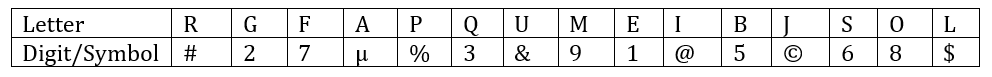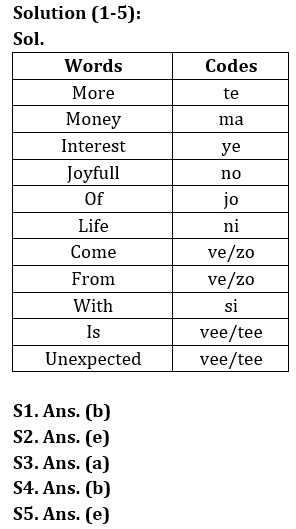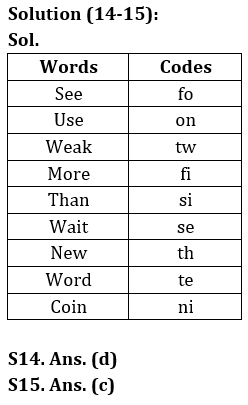Latest Banking jobs   »

# Reasoning Ability Quiz For LIC AAO Mains 2023-10th March

Direction (1-5): Study the following information carefully to answer the questions.
In a certain code,
‘Money interest more’ is written as ‘ma te ye’.
‘More of joyfull’ is written as ‘te jo no’.
‘Joyfull come from money’ is written as ‘no ve ma zo’.
‘Life with more of joyfull’ is written as ‘si ni te no jo’.
‘Life is unexpected’ is written as ‘vee ni tee’.

Q1. What is the code for ‘life’?
(a) si
(b) ni
(c) ti
(d) no
(e) Either ‘ni’ or ‘si’

Q2. Which of the following represents ‘life lot of joyfull’?
(a) ma te jo no
(b) si te jo no
(c) te jo no ye
(d) te no jo ke
(e) ni te jo no

Q3. Which of the following may represent ‘life with more of sorrow’?
(a) ni si jo te shi
(b) ni si jo te ve
(c) ni si jo te tee
(d) si ni jo te zo
(e) si ni zo yo ma

Q4. What does ‘zo’ stand for?
(a) Either ‘life’ or ‘with’
(b) Either ‘come’ or ‘from’
(c) Either ‘come’ or ‘with’
(d) Either ‘come’ or ‘life’
(e) None of these

Q5. Which of the following may represent ‘money with more of joyfull’?
(a) si ni te jo ma
(b) te jo ni si ye
(c) si ni te jo no
(d) so si ni jo te
(e) ma si jo no te

Directions (6-10): In each of the following below is given a group of letters followed by four combinations of digits/symbols numbered (a), (b), (c) and (d). You have to find out which of the combinations correctly represents the group of letters based on the following coding system and mark the number of that combination as the answer. If none of the four combinations correctly represents the group of letters, mark (e), i.e. ‘None of these’, as the answer-

Note: More than one condition may apply.Conditions:
(i) If first letter is vowel and last letter is consonant then both are coded with the code of the consonant.
(ii) If both the 2nd and the last letter is vowel, then their codes are to be interchanged.
(iii) If the second letter is a consonant and the 2nd last letter is a vowel, both are to be coded as the code for the vowel.
(iv) If both 1st and fifth letter is consonant then both are coded as the code of third letter.
(v) If only one condition is applied among the above given, then the code of first letter is interchanged with code of second letter and third letter code interchanged with 4th letter and so on after that applied condition.

Q6. URBSAQ
(a) 3#65#3
(b) 3µ56µ3
(c) &56\$33
(d) &µ65µ&
(e) None of these

Q7. LIFPRE
(a) 7177%@
(b) 771%7@
(c) 717%@7
(d) 717%7@
(e) None of these

Q8. EUMRJA
(e) None of these

Q9. MJGLBF
(a) %3%6%7
(b) 36%%7@
(c) 3#6%7@
(d) 3%6%7#
(e) None of these

Q10. LRQBJS
(a) %53328
(b) #33536
(c) #35363
(d) @3567@
(e) None of these

Direction (11-13): Study the following information carefully and answer the questions accordingly.
In an artificial language,
‘Gourmet intrinsic appointed’ is coded as – ‘%L &M &K’
‘Castigate censure blatant’ is coded as – ‘%O %V %Z’
‘Calculate overcome contrive’ is coded as – ‘&E %Z %L’

Q11. What will be the code for ‘Abound announce’?
(a) %V &E
(b) &K %Z
(c) %D &F
(d) &M &Y
(e) None of the above

Q12. Which of the following word will be coded as ‘%Z %L’?
(a) Practical issue
(b) Making formal
(c) Praise problem
(d) Prevent smoke
(e) None of the above

Q13. If the code for ‘Contrive empty’ is ‘%R, ____. Which of the following code will fill the blank space?
(a) %V
(b) %F
(c) &P
(d) &G
(e) None of these

Directions (14-15): Answer the questions based on the information given below.
In a certain code of language,
‘See use weak more’ is coded as ‘tw on fo fi’
‘Wait new than more’ is coded as ‘si fi se th’
‘Use word coin than’ is coded as ‘ni si te on’
‘Weak wait use coin’ is coded as ‘tw on ni se’

Q14. What is the code of ‘coin word’?
(a) on tw
(b) te th
(c) fi si
(d) ni te
(e) None of these

Q15. Which of the following word is coded as ‘tw’?
(a) See
(b) Use
(c) Weak
(d) Than
(e) None of these

SolutionsSolution (6-10):
S6. Ans(b)
Sol. Condition (i) and (iii) is applied
S7. Ans(d)
Sol. Condition (ii) and (iv) is applied
S8. Ans(a)
Sol. Condition (ii) and (v) is applied
S9. Ans(e)
Sol. Condition (iv) and (v) is applied
S10. Ans(c)
Sol. Condition (iv) and (v) is applied

Solution (11-13):
S11. Ans. (d)
Sol. Logic: Symbol: (i) If first letter is consonant then % is used
(ii) If the first letter is vowel, then & is used
Letter: Second letter of the word changed to opposite letter
The code for ‘Abound announce’ – ‘&M &Y’

S12. Ans. (b)
Sol. Logic: Symbol: (i) if first letter is consonant then % is used
(ii) if the first letter is vowel, then & is used
Letter: Second letter of the word changed to opposite letter
Note: Such type of questions is solved with the help of elimination method. We must check all the options one by one.
The code for ‘Making formal’ – %Z %L

S13. Ans. (c)
Sol. Logic: Symbol: (i) If first letter is consonant then % is used
(ii) If the first letter is vowel, then & is used
Letter: Second letter of the word changed to opposite letter
The code for ‘Contrive empty’ is ‘%R, &P’.## FAQs

### When will the LIC AAO Mains exam be held?

LIC AAO Mains exam will be held on 18 March

#### Congratulations!Union Budget 2023-24: Free PDF